# Prime Spiral #2

Today's post was inspired by a YouTube video, Why do prime numbers make these spirals?, on the channel 3Blue1Brown, created by Grant Sanderson. In my opinion this is the best math channel on YouTube. He has beautiful graphics and superb exposition. I recommend you take a look, if you haven't already.

My 2015 post Prime Spiral was about a completely different prime spiral discovered by Stan Ulam.

### Contents

#### Spiral Polar Plots

The following figure shows the plotting scheme. We are using polar coordinates, $r, \theta$, with $\theta$ measured in radians.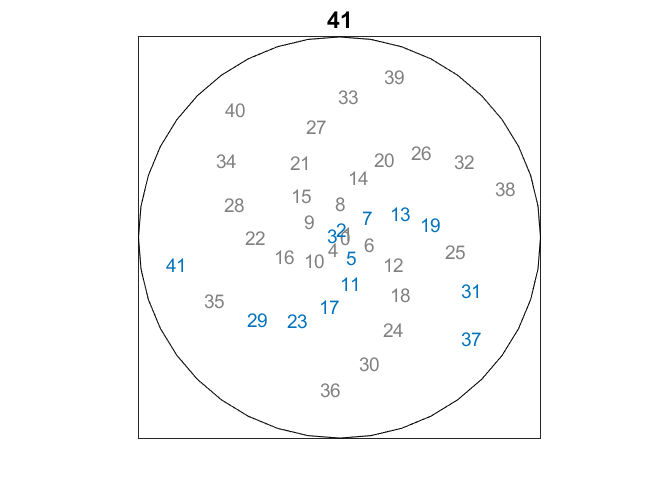Each integer $n$ is plotted at the point where both $r$ and $\theta$ are equal to $n$. So, for example, "6" is placed 6 units from the origin, at an angle of 6 radians from the positive x-axis. Since 6 is a little less than $2\pi$, this puts 6 in the fourth quadrant, a little bit under the x-axis. Moreover 12 is near 6 and 18 near 12. The multiples of 6 lie on an arc that is turning clockwise, orthogonal to the counterclockwise spiral of the underlying numbering scheme. There are six arcs altogether because 6 is almost $2\pi$.

The primes are shown in blue. They occupy only two of the six arms because the numbers on the other four arms are all divisible by 2 or 3.

#### r <= 100

Here is a plot of the integers up to 100. The six clockwise spirals are clearly visible. The two arms with the blue primes stand out, but the only property of prime numbers that is relevant is that they are not divisible by 2 and 3, the factors of 6.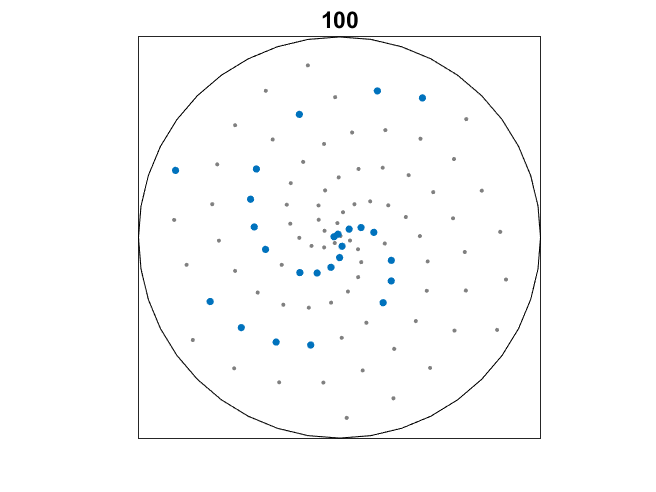#### r <= 300

Here is a plot of the integers up to 300. It's a bit jumbled. The six arms are less visible, but do you see anything taking their place?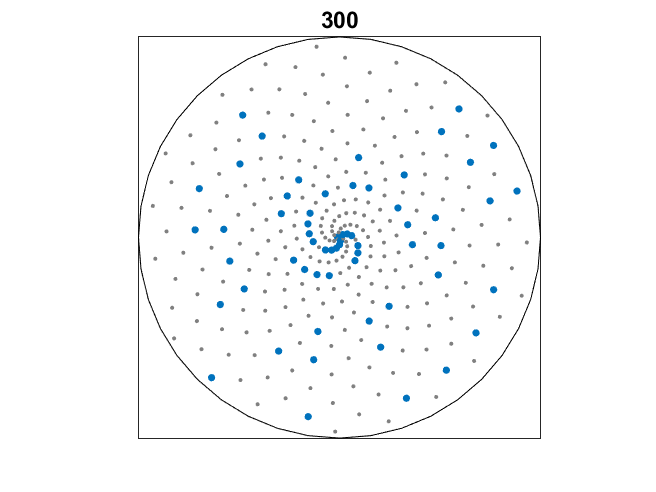#### r <= 600

The numbers up to 600. Now a new family of spirals is visible. There are 44 of them. That's because 44 is close to a multiple of $2\pi$. This fact is better known as 22/7 is a fair approximation to $\pi$. The spirals are turning counterclockwise because 44 is greater than $7\pi$ whereas the earlier spirals are turning clockwise because 6 is less than $2\pi$.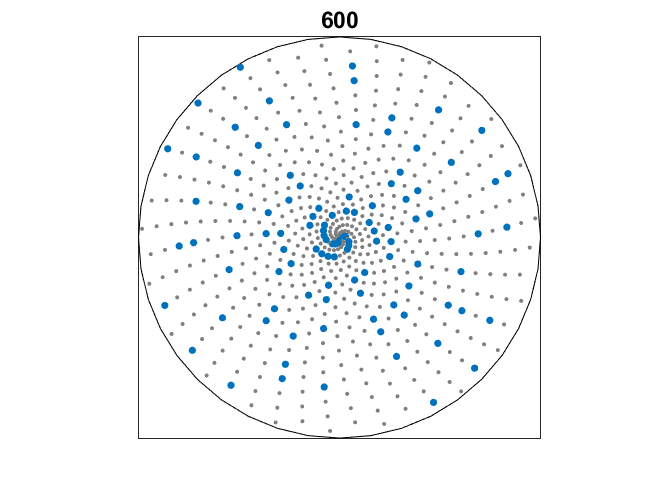#### r <= 2000

The integers up to 2000. The 44 arcs are dominant. Homework: which arms contain the primes?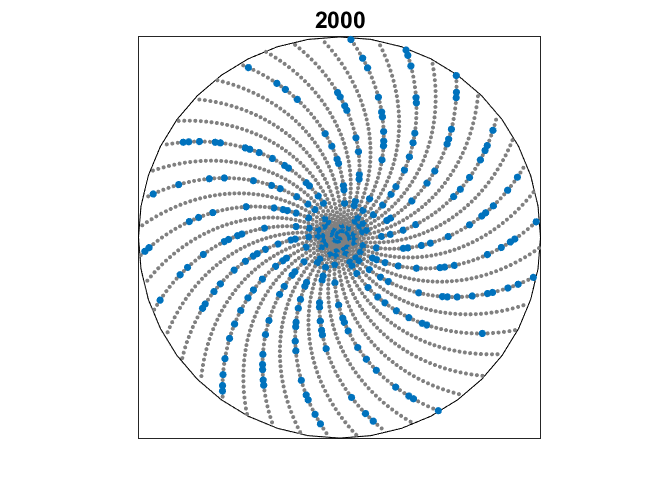#### r <= 20000

It was getting crowded, so now it's just the blue points, the primes, up to 20,000. What is that big, white swath?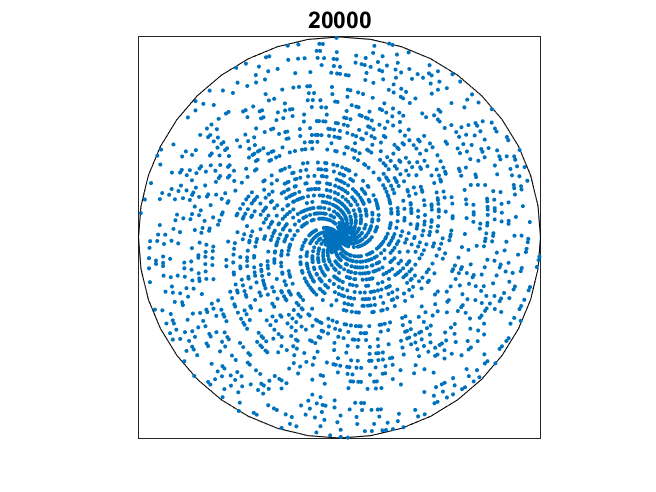#### r <= 100000

The primes less than 100,000. New structures emerge. These radial arms appear because 355/115 is a remarkably good approximation to $\pi$. We are finally seeing evidence of a deep fact about the primes, Dirichlet's theorem on the distribution of primes among residue classes. See Grant Sanderson's video for the details.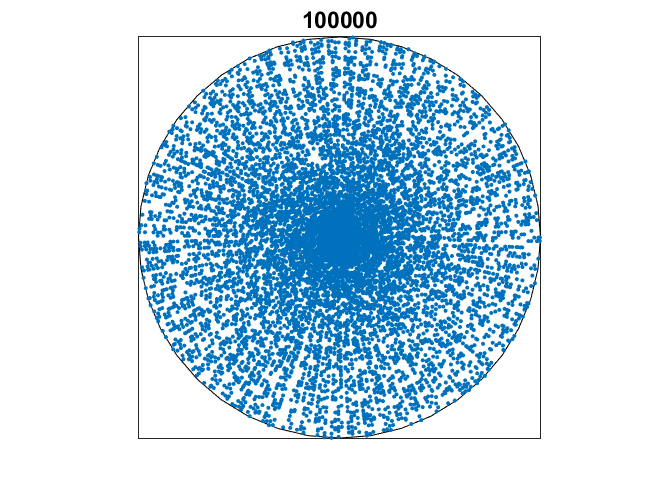#### prime_spiral_2.m

Here an animated gif showing my app prime_spiral_2 in action. It automatically zooms in and out continuously, or you can drive it yourself with a scroll bar. This app is included in release 4.7 of Cleve's Laboratory. Send me email if you would like a standalone copy.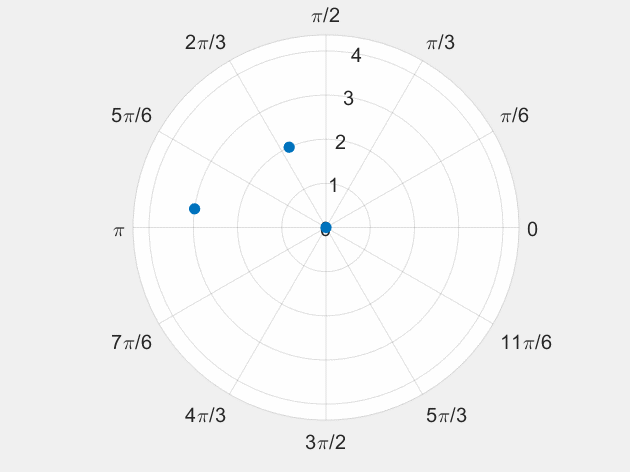Published with MATLAB® R2018b

|